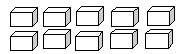Name: ___________________Date:___________________

 Email us to get an instant 20% discount on highly effective K-12 Math & English kwizNET Programs!

### Grade 3 - Mathematics1.18 Multiplication Review Test

 Q 1: 12 x 0 = Answer: Q 2: 2 x 33 = Answer: Q 3: 77 x 7 = Answer: Q 4: Use the figure to find 5 times 2.111012 Q 5: What is the multiplication form shown in the figure?2 x 4 = 82 x 4 = 102 x 5 = 8 Q 6: 8 x 2 =161014 Q 7: Sue has four baskets with three eggs in each. How many eggs in all?12734 Q 8: 8 x 8 =647210488 Question 9: This question is available to subscribers only! Question 10: This question is available to subscribers only!

#### Subscription to kwizNET Learning System costs less than \$1 per month & offers the following benefits:

• Unrestricted access to grade appropriate lessons, quizzes, & printable worksheets
• Instant scoring of online quizzes
• Progress tracking and award certificates to keep your student motivated
• Unlimited practice with auto-generated 'WIZ MATH' quizzes